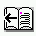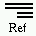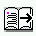DOC HOME SITE MAP MAN PAGES GNU INFO SEARCH PRINT BOOK

### Berkeley DB Reference Guide:Access Methods### Partial record storage and retrieval

It is possible to both store and retrieve parts of data items in all Berkeley DB access methods. This is done by setting the DB_DBT_PARTIAL flag in the DBT structure passed to the Berkeley DB method.

The DB_DBT_PARTIAL flag is based on the values of two fields of the DBT structure: dlen and doff. The value of dlen is the number of bytes of the record in which the application is interested. The value of doff is the offset from the beginning of the data item where those bytes start.

For example, if the data item were ABCDEFGHIJKL, a doff value of 3 would indicate that the bytes of interest started at D, and a dlen value of 4 would indicate that the bytes of interest were DEFG.

When retrieving a data item from a database, the dlen bytes starting doff bytes from the beginning of the record are returned, as if they comprised the entire record. If any or all of the specified bytes do not exist in the record, the retrieval is still successful and any existing bytes are returned.

When storing a data item into the database, the dlen bytes starting doff bytes from the beginning of the specified key's data record are replaced by the data specified by the data and size fields. If dlen is smaller than size, the record will grow, and if dlen is larger than size, the record will shrink. If the specified bytes do not exist, the record will be extended using nul bytes as necessary, and the store call will still succeed.

The following are various examples of the put case for the DB_DBT_PARTIAL flag. In all examples, the initial data item is 20 bytes in length:

ABCDEFGHIJ0123456789

1. ```size = 20
doff = 0
dlen = 20
data = abcdefghijabcdefghij

Result: The 20 bytes at offset 0 are replaced by the 20 bytes of data;
that is, the entire record is replaced.

ABCDEFGHIJ0123456789 -> abcdefghijabcdefghij
```

2. ```size = 10
doff = 20
dlen = 0
data = abcdefghij

Result: The 0 bytes at offset 20 are replaced by the 10 bytes of data;
that is, the record is extended by 10 bytes.

ABCDEFGHIJ0123456789 -> ABCDEFGHIJ0123456789abcdefghij
```

3. ```size = 10
doff = 10
dlen = 5
data = abcdefghij

Result: The 5 bytes at offset 10 are replaced by the 10 bytes of data.

ABCDEFGHIJ0123456789 -> ABCDEFGHIJabcdefghij56789
```

4. ```size = 10
doff = 10
dlen = 0
data = abcdefghij

Result: The 0 bytes at offset 10 are replaced by the 10 bytes of data;
that is, 10 bytes are inserted into the record.

ABCDEFGHIJ0123456789 -> ABCDEFGHIJabcdefghij0123456789
```

5. ```size = 10
doff = 2
dlen = 15
data = abcdefghij

Result: The 15 bytes at offset 2 are replaced by the 10 bytes of data.

ABCDEFGHIJ0123456789 -> ABabcdefghij789
```

6. ```size = 10
doff = 0
dlen = 0
data = abcdefghij

Result: The 0 bytes at offset 0 are replaced by the 10 bytes of data;
that is, the 10 bytes are inserted at the beginning of the record.

ABCDEFGHIJ0123456789 -> abcdefghijABCDEFGHIJ0123456789
```

7. ```size = 0
doff = 0
dlen = 10
data = ""

Result: The 10 bytes at offset 0 are replaced by the 0 bytes of data;
that is, the first 10 bytes of the record are discarded.

ABCDEFGHIJ0123456789 -> 0123456789
```

8. ```size = 10
doff = 25
dlen = 0
data = abcdefghij

Result: The 0 bytes at offset 25 are replaced by the 10 bytes of data;
that is, 10 bytes are inserted into the record past the end of the
current data (\0 represents a nul byte).

ABCDEFGHIJ0123456789 -> ABCDEFGHIJ0123456789\0\0\0\0\0abcdefghij
```